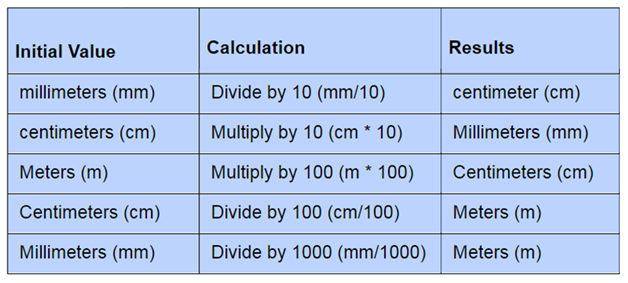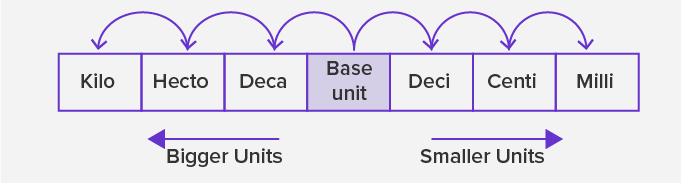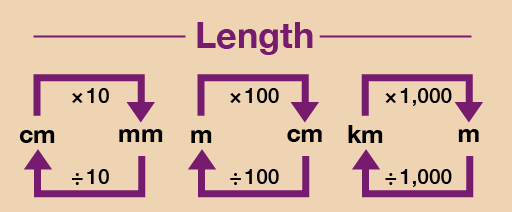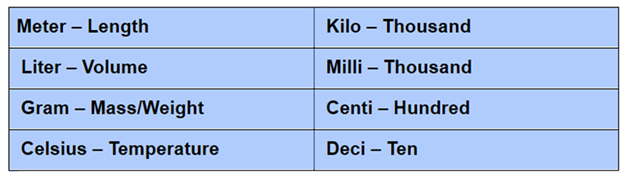# Units of Measurement – Definition, Conversion, Examples | Metric Units of Length, Mass, Volume, Time

In the metric system of measurement, the meter is the basic unit of length, a gram is the basic unit of mass and liter is the basic unit of capacity.  We can use a centimeter(cm) to measure the length. Centimeter and Millimeter are very small units to measure the length, so we use another unit called meters.

Learn completely about the Units of a Measurement- Definition, Units Conversion, Prefix for Length, Time, Weight, and Volume or Capacity. Get to know the Importance of SI Units, Solved Examples on How to Convert one unit to another, etc.

## Metric System – Introduction

The French are widely credited with originating the metric system of measurement, the system is officially adopted in 1795. It was originated in the year 1799. Metric System is basically a system used for measuring distance, length, volume, weight, and temperature. The term metric system is used as another word for SI or the international system of units.  Based on three basic units we can measure almost everything in the world, those are M- Meter, used to measure the length, Kg- Kilogram, used to measure the mass, and S- Second, used to measure time.

### Units of Measurement – Definition

The SI system, also called the metric system, is used around the world. SI units stand for standard International System of the units. Seven basic units in the SI  system, give proper definitions for meter, kilogram, and the second. It also specifies and defines remaining four different  units:

1. Kelvin(K)- used to measure the Temperature

2. Ampere(A)- used to measure the Electric current

3. Candela(cd)- used to measure the Luminous Intensity

4. Mole(mol)- used to measure  the Material Quantity

### Units of Measurement Conversion

To convert among units in the metric system, identify the unit that you have, the unit that you want to convert to, and then count the number of units between them. Some units are connected with each other by the following relation:

1 Kilometer (km) = 1000 meter (m)

1 meter (m) = 100 centimeter (cm)

1 centimeter (cm) =  10 millimeter (mm)### Metric Units Prefix

A metric prefix is a unit prefix that precedes a basic unit of measure to indicate a multiple or submultiple of the unit. To convert from one unit to another within the metric system usually means moving a decimal point. you can convert within the metric system relatively easily by simply multiplying or dividing the number by the value of prefix.

In order to remember the proper movements of units, arrange the prefixes from the largest to the smallest.Now, let us discuss some of the units for length, weight, volume, time.

### Units of Measurement Length

The most common unit used to measure the length are as follows. Centimeters and millimeters are very small to measure the length so, we use another unit that is the meter (m). Even meter is too small when we measure the distance between two cities, we use kilometers (km).Kilometer (km) Hectometer (hm) Decameter (dam) Meter (m) Decimeter (dm) Centimeter (cm) Millimeter (mm) 1000 100 10 1 1/10 1/100 1/1000

### Units of Measurement for Volume or Capacity

A liter is a metric unit of volume. The most common units used to measure the capacity or volume of any object are as follows:

1 liter (l) = 1000 milliliters (ml)

 Kiloliter (kl) Hectoliter (hl) Decaliter(dal) Liter (l) Deciliter (dl) Centiliter(cl) Milliliter(ml) 1000 100 10 1 1/10 1/100 1/1000

### Units of Measurement for Weight

To measure the weight of the compound, we can use a smaller unit called milligrams. The most common units to measure the weight of any object are as follows:

1 kilograms (kg) = 1000 grams (gm)

1 grams (gm) = 1000 milligrams (mg)

1 kilograms (kg) = 1000 × 1000 milligrams (mg) = 1,000,000 milligrams (mg)

 Kilogram (kg) Hectogram (hg) Decagram (dag) Gram (g) Decigram (dg) Centigram(cg) Milligram (mg) 1000 100 10 1 1/10 1/100 1/1000

### SI Unit of Measurement for Time

The SI unit for the period, as for all the measurements of time, is the Second. The other units of Time are minute, hour, day, week, month, year, and century. Now let us discuss some other units of time.

1 minute = 60 seconds

1 hour = 60 minutes

1day = 24 hours

1 week = 7 days

1 month = 30 or 31 days

NOTE: February has 28 days, but in leap year February has 29 days.

1 year = 12 hours or 365 days (in a leap year 366 days)

### Importance of Standard Unit of Measurement

We need standard units for measurement, to make our judgment more reliable, accurate, and uniformity.  It is important because it allows scientists to compare data and communicate with each other about their results. To avoid confusion when measuring, scientists use a shared system of measurement called the international system of units (SI).### Units of Measurement Examples

Example 1: Convert  248 centimeters to meters?

Solution:

We know that, 1 cm = 0.01 mThus , 248 cm = 248 x 0.01 = 2.48 m

now , 248 cm = 2. 48 m

Therefore, 248 cm is equivalent to 2.48 m.

Example 2:

Convert  2000 grams to kilograms?

Solution:

We know that, 1 gram = 0.001 grams

Thus , 2000 grams = 2000 x 0.001 = 2 kilogram

2000 grams = 2 kilograms

Therefore, 2000 grams is equivalent to 2 kilograms.

Example 3:

Convert 20 kiloliters to liters?

Solution:

We know that 1 kiloliter = 1000 liters

Thus, 20 litres = 20 x 1000 litres = 20000 liters

20 kiloliters = 20000 liters

Therefore, 20 kiloliters are equivalent to 20000 liters.

Example 4:

Convert  150 kg to milligrams?

Solution:

We know that, 1 gram = 1000 milligrams and 1 kg = 1000 grams

So, first we convert the kg to g as :

1 kg = 1000 g

Therefore,  150 kg = 150 x 1000 g = 150,000 grams

Now, converting g to mg:

1 g = 1000 mg

Therefore , 150,000 g = 150,000 x 1000 mg = 250,000,000 mg.

#### FAQ’S on Units of Measurements

1.  What are the base units for Length, Weight, and Volume in a Metric System?

The base units for length, weight, and volume in a metric system are meters, grams, and liters respectively.

2. Mention the US Standard Units for Length, Weight, and Volume?

In US systems, the units used are:

• Distance or length in miles, yards, feet, inches
• Mass or weight in pounds, tons, ounces
• Capacity or volume in cups, gallons or quarts, pints, fluid ounces.

3. What are the advantages of using a Standard Unit of Measurement?

The advantage of the SI unit is, it has only one unit for each quantity. suppose the one and only SI unit of length is the meter (m).

4. Why do we use Measurement?

Measurements require tools and provide scientists with a quantity. A quantity describes how much of something there is or how many there are.

5. What is a Standard Unit?

Standard units are the units we usually use to measure the weight, length, and volume of the objects.

Scroll to Top